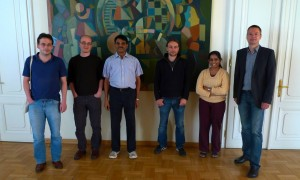# Mini-Workshop

## Mini-Workshop on Logic, Proofs and Algorithms

The VCLA, the Wolfgang-Pauli-Institute and the Austrian Agency for International Cooperation in Education and Research hosted a mini-workshop on logic, proofs and algorithms organized by Stefan Szeider.

 DATE: Wednesday, June 6, 2012 TIME: 10:00 – 16:00 VENUE: Lecture room 2, Theresianumgasse 27, 1040 Vienna## Schedule

You can find directions to the lecture room here.

Morning Session 10:00-12:30

Venkatesh Raman
Barnaby Martin
Moritz Müller

Lunch 12:30-14:00

Restaurant Ischia, Mozartplatz 1, 1040 Vienna

Please write an e-mail to Katarina Jurik until Tuesday, June 5th if you would like to attend lunch together with the group.

Afternoon Session: 14:00-16:00

Friedrich Slivovsky
Neeldhara Misra

## Abstracts

#### Barnaby Martin

Durham University, UK

Title: Parameterized Proof Complexity

Abstract: The origins of Proof Complexity lie in the program of Cook and Reckow, who aimed to separate P and NP (actually, coNP and NP) by proving that no propositional proof system is polynomially bounded. Having failed to obtain such a general result, students of Proof Complexity have instead focused on proving that stronger and stronger proof systems are not polynomially bounded. Such lower bounds are known for systems such as Resolution and Bounded-depth Frege.

We propose a proof-theoretic approach for gaining evidence that certain parameterized problems are not fixed-parameter tractable. We consider proofs that witness that a given propositional CNF formula cannot be satisfied by a truth assignment that sets at most k variables to true, considering k as the parameter (we call such a formula a parameterized contradiction). One could separate the parameterized complexity classes FPT and W by showing that there is no fpt-bounded proof system, i.e., that there is no proof system that admits proofs of size f(k).n^{O(1)} where f is a computable function and n represents the size of the propositional formula.

We survey results in the area, taking in the parameterized versions of tree-Resolution, Resolution and bounded-depth Frege.

#### Moritz Müller

University of Vienna, Austria

Title: Parameterizations of strong proof systems.

Abstract: we introduce a new notion of parameterized proof system and study parameterized versions of classical strong proof systems such as Extended Frege, Renaming Frege or Substitution Frege. We are interested in their comparative strength with respect to suitable notions of parameterized simulations.

#### Venkatesh Raman

Institute of Mathematical Sciences, Chennai, India

Title: Fixed parameter tractability of satisfying beyond the number of variables

Abstract: Given a boolean CNF formula, let m be the size of the maximum matching in the natural variable-clause incidence bipartite graph corresponding to the formula. It is easy to show that one can satisfy at least m clauses of the formula. We show that testing whether one can satisfy at least m + k clauses of the formula is fixed-parameter tractable, where k is the parameter.

#### Neeldhara Misra

Institute of Mathematical Sciences, Chennai, India

Title: Separators with Non-Hereditary Properties

Abstract: We investigate the problem of finding small s–t separators that induce graphs having certain properties. It is known that finding a minimum clique s–t separator is polynomial-time solvable (Tarjan 1985), while for example the problems of finding a minimum s–t separator that is a connected graph or an independent set are fixed-parameter tractable (Marx, O’Sullivan and Razgon, manuscript). We explore how these results generalize, for instance, when we require that the separator has diameter at most d (the clique being the special case when d=1) or when the separator is required to be c-connected (the connected separators being the special case when c=1). In general, these problems turn out to be W-hard, although it is curious that the problem of finding a 2-connected separator is FPT.

It also turns out that on graphs of bounded degree, the problem of finding a minimum s-t separator with any decidable property is FPT. After an overview of the results, we will discuss some specific algorithms.

#### Friedrich Slivovsky

Vienna University of Technology, Austria

Title: Backdoor sets of QBFs and resolution-path dependencies

Abstract: The concept of backdoor sets can be generalized from propositional satisfiability to quantified boolean formulas by adding the requirement that backdoor sets must be closed with respect to dependencies among quantified variables (Samer & Szeider 2009). Dependency schemes are a general framework for representing the relevant kind of dependencies. Since it is generally intractable to determine dependencies exactly, we instead investigate the problem of computing a dependency scheme that may include false positives. Provided that such a scheme can be computed in polynomial time, evaluating QCNF formulas is fixed-parameter tractable when parameterized by the size of a smallest strong backdoor set (with respect to the classes QHORN or Q2CNF). Among the schemes proposed so far, resolution path dependencies introduce the fewest spurious dependencies. We describe an algorithm that detects resolution-path dependencies in linear time, resolving a problem posed by Van Gelder (CP 2011). (Joint work with Stefan Szeider)